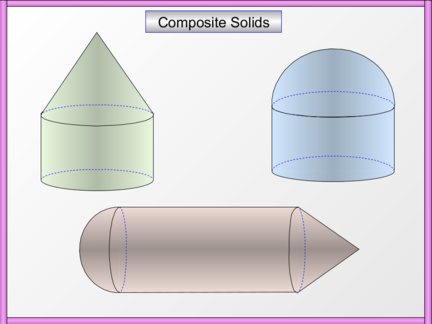# Volume of Composite SolidsContributed by:In this pdf, we will learn how to calculate the volume of Composite Solids. To calculate the volume of a composite solid, simply split it into smaller solids and calculate their separate volumes. The volumes of each of the individual solids are then added together to give the total volume of the composite solid.
1. Composite Solids
2. Example Question 1 Composite Solids
An aeronautical engineer designs a small component part made of copper, that is
to be used in the manufacturer of an aircraft. The part consists of a cone that sits
on top of a cylinder as shown in the diagram below. Find the volume of the part.
Volume of cone = 1/3 r2h
= 1/3 x  x 42 x 9
9 cm
= 48 cm3
8 cm
Vol/Cap
Volume of cylinder = r2h
=  x 42 x 6
6 cm = 96 cm3
Total volume = 48 + 96 = 144 cm3
3. Example Question 2 Composite Solids
The shape below is composed of a solid metal cylinder capped with a solid metal
hemi-sphere as shown. Find the volume of the shape. (to 3 sig fig)
Volume of hemi-sphere = 2/3 r3
= 2/3 x  x 33
= 18 m3
6m
Volume of cylinder = r2h
4m =  x 32 x 4
= 36 m3
Total volume = 18 + 36 = 54 m3
= 170 m3
4. Example Question 3 Composite Solids
The diagram below shows a design for a water tank. The water tank consists of a
cylinder capped with a hemi-spherical dome. Find the capacity of the water tank.
Capacity of hemi-sphere = 2/3 r3
= 2/3 x  x 33
6m = 18 m3
Capacity of cylinder = r2h
5m =  x 32 x 5
= 45 m3
1 000
1 000 000cm
cm3 3 Total capacity = 18 + 45 = 63 m3
= 63 000 000 cm3
10 cm
100 cm 1 = 63 000 litres
litre
10
100cm
cm = 200 000 litres (2 sig fig)
10 cm
100 cm
5. Example Question 4 Composite Solids
A solid shape is composed of a cylinder with a hemi-spherical
14 cm base and a conical top as shown in the diagram. Calculate the
volume of the shape. (answer to 2 sig fig)
Volume of cone = 1/3 x r2h
= 1/3 x  x 62 x 14
= 168 cm3
Volume of cylinder = r2h
=  x 62 x 40
40 cm
= 1440 cm3
Volume of hemi-sphere = 2/3 r3
= 2/3 x  x 63
= 144 cm3
12 cm
Total volume = 168 + 1440 + 144 = 1752 cm3
= 5500 cm3
6. Question 1 Composite Solids
An aeronautical engineer designs a small component part made of copper, that is
to be used in the manufacturer of an aircraft. The part consists of a cone that sits
on top of a cylinder as shown in the diagram below. Find the volume of the part.
Volume of cone = 1/3 r2h
= 1/3 x  x 52 x 12
12 cm
= 100 cm3
Volume of cylinder = r2h
10 cm
=  x 52 x 6
6 cm = 150 cm3
Total volume = 100 + 150 = 250 cm3
7. Question 2 Composite Solids
The shape below is composed of a solid metal cylinder capped with a solid metal
hemi-sphere as shown. Find the volume of the shape. (to 2 sig fig)
Volume of hemi-sphere = 2/3 r3
= 2/3 x  x 93
= 486 cm3
18 cm
Volume of cylinder = r2h
10 =  x 92 x 10
cm
= 810 m3
Total volume = 486 + 810 = 1296 cm3
= 4100 cm3
8. Question 3 Composite Solids
The diagram below shows a design for a water tank. The water tank consists of a
cylinder capped with a hemi-spherical dome. Find the capacity of the water tank.
Capacity of hemi-sphere = 2/3 r3
= 2/3 x  x 63
12 m = 144 m3
Capacity of cylinder = r2h
10m =  x 62 x 10
= 360 m3
1 000
1 000 000cm
cm3 3 Total capacity = 144 + 360 = 504 m3
= 504 000 000 cm3
10 cm
100 cm 1 = 504 000 litres
litre = 1 580 000 litres (3 sig fig)
10
100cm
cm
10 cm
100 cm
9. Question 4 Composite Solids
A solid shape is composed of a cylinder with a hemi-spherical
9 cm base and a conical top as shown in the diagram. Calculate the
volume of the shape. (answer to 2 sig fig)
Volume of cone = 1/3 x r2h
= 1/3 x  x 32 x 9
= 27 cm3
Volume of cylinder = r2h
20 cm =  x 32 x 20
= 180 cm3
Volume of hemi-sphere = 2/3 r3
= 2/3 x  x 33
= 18 cm3
6 cm
Total volume = 27 + 180  + 18 = 225  cm3
= 710 cm3
10. Example Questions Surface Area
Worksheets
11. Questions Surface Area
12. Example Questions Volume/Capacity
13. Questions Volume/Capacity